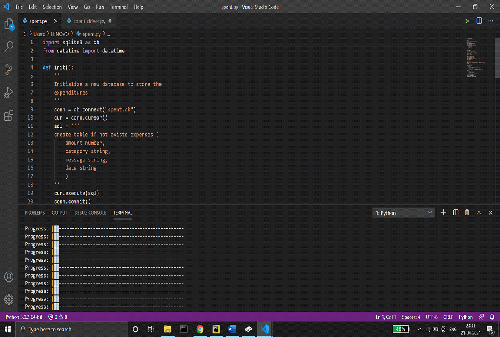how do you create/ make an expense tracker in python

## how do you create/ make an expense tracker in python

Hello everyone, in this article we will see how do you create/ make an expense tracker in python with source code so let’s see :

### Introduction about expense tracker in python :

Expense tracking is important for the financial health of your personal or professional budget.
You need to keep a record of your daily expenses and stocks in a proper format so that you can analyze all your expenditures efficiently and correctly.

#### How about an application that does all this work for you and you just need to feed the information?

So, understanding the importance of the application, we are here to give you a nice explanation and implementation of the same. SQLite is a C-language library that implements a small, fast, self-contained, high-reliability, full-featured, SQL database engine. SQLite is the most used database engine in the world. SQLite is built into all mobile phones and most computers and comes bundled inside countless other applications that people use every day.
A date in Python is not a data type of its own, but we can import a module named datetime to work with dates as date objects.

### Installation for expense tracker

You can install docopt module in various ways, pip is one of the best ways to install docopt.
\$pip install docopt
The tabulate() method is a method present in the tabulate module which creates a text-based table output inside the python program using any given inputs. It can be installed using the below command
pip install tabulate

### Source code to create/ make an expense tracker in python

this source code help you to create expense tracker in simple way

``````Spent.py
import sqlite3 as db
from datetime import datetime

def init():
'''
Initialize a new database to store the
expenditures
'''
conn = db.connect("spent.db")
cur = conn.cursor()
sql = '''
create table if not exists expenses (
amount number,
category string,
message string,
date string
)
'''
cur.execute(sql)
conn.commit()

def log(amount, category, message=""):
'''
logs the expenditure in the database.
amount: number
category: string
message: (optional) string
'''
date = str(datetime.now())
data = (amount, category, message, date)
conn = db.connect("spent.db")
cur = conn.cursor()
sql = 'INSERT INTO expenses VALUES (?, ?, ?, ?)'
cur.execute(sql, data)
conn.commit()

def view(category=None):
'''
Returns a list of all expenditure incurred, and the total expense.
If a category is specified, it only returns info from that
category
'''
conn = db.connect("spent.db")
cur = conn.cursor()
if category:
sql = '''
select * from expenses where category = '{}'
'''.format(category)
sql2 = '''
select sum(amount) from expenses where category = '{}'
'''.format(category)
else:
sql = '''
select * from expenses
'''.format(category)
sql2 = '''
select sum(amount) from expenses
'''.format(category)
cur.execute(sql)
results = cur.fetchall()
cur.execute(sql2)
total_amount = cur.fetchone()

Spent_driver.py
usage = '''
Expense Tracker CLI.
Usage:
spent_driver.py init
spent_driver.py view [<view_category>]
spent_driver.py <amount> <category> [<message>]
'''

from docopt import docopt
from spent import *
from tabulate import tabulate

args = docopt(usage)

if args['init']:
init()
print("User Profile Created")

if args['view']:
category = args['<view_category>']
amount, results = view(category)
print("Total expense: ", amount)
print(tabulate(results))

if args['<amount>']:
try:
amount = float(args['<amount>'])
log(amount, args['<category>'], args['<message>'])
except:
print(usage)``````

Summary :